## Multivariate distance matrix

In this section of similarity tutorial, I will give a simple but comprehensive example on how we can aggregate mixed type of data (multivariate data) up to N dimension. Here I put 4 objects (family) and 5 multivariate features (binary, nominal, ordinal, and quantitative measurement scale). The goal is to transform this data into aggregated distance matrix.

Here is step by step on how to aggregate multivariate distances:

1. Convert data into coordinate based on measurement scale
2. Determine distance matrix for each features variable based on coordinate
3. Normalize the distance matrix into range of [0, 1]
4. Aggregate the distance matrix

Once you get the distance matrix, you may use the distance matrix for various purposes such as clustering (i.e. K means clustering) and data reduction (multidimensional scaling, Principle component analysis) and so on.

Example:

Suppose we have the following data from Park visitors (extracted from my Park study, the actual data may include hundreds of families and hundreds of variables). Here is the meaning of each variable

• Family is the object that we want to measure the distance. A, B, C, and D are their name.
• Time is a quantitative data type , measured in minutes. It is the activity time of the family in the park
• Mode is nominal data type consist of four choice of mode to go to park: (1) walk, (2) car, (3) cycling, (4) bus. The choice is mutually exclusive, that is only one mode for one family.
• Activity is nominal data type consist of 6 choices of activity in the park: (1) sport, (2) picnic, (3) reading, (4) walk (including with the dog), (5) meditation, (6) jog. The choices are multiple choices, that one family may have several activities in the park.
• Satisfaction is ordinal scale with 5 values: -2 = Very dissatisfied, - 1 = dissatisfied, 0 = indifference, 1 = satisfied, 2 = Very satisfied. It measures family satisfaction toward the park's services.
• Playground is binary scale (Yes or No) about the existence of children playground

The data is show in this table

 Family Time Mode Activity Satisfaction Playground A 30 1 1, 2, 3 2 Y B 30 3 4,6 1 N C 60 2 1, 2 2 Y D 45 1 5 -1 Y

#### 1. Transform data into coordinate

First, we need to transform this data into coordinate. Each family will be a point in 6 dimensional features space. To transform the data into coordinate, we need to consider each features data type. If the data is quantitative (i.e. Time ), we don't need to change anything. If the data is binary (i.e. Playground ), we convert it into 0 and 1.

If the data is ordinal (i.e. Satisfaction and Green ), we get the rank and normalize the rank into range [0, 1].

Here is the conversion of rank and normalized description of Satisfaction featureFor instance, family D has satisfaction of -1, the normalized rank is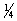Variable Mode is nominal with four mutually exclusive values of (1) walk, (2) car, (3) cycling, (4) bus. Because they are mutually exclusive, it would be better if we assign each value of category into several binary dummy variables. We have number of dummy variable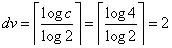and we name it (DV1, DV2). Here is the conversion table of nominal variable Mode (with four values of category):

 Mode 1 2 3 4 Meaning walk car cycling Bus DV1 0 1 0 1 DV2 0 0 1 1

For instance, Family D is using is walking to go to park, the mode = 1, now converted into coordinate as (DV1, DV2) = (0,0).

Variable Activity is a nominal scale with multiple choices value of (1) sport, (2) picnic, (3) reading, (4) walk (including with the dog), (5) meditation, (6) jog. Because one family can have several choices, we must assign each value of category into a single binary variable. Thus, we have six internal coordinate of this nominal variable. The internal coordinate represents the six categories: (sport, picnic, reading, walk, meditation, jog). For instance, family A has three activities, thus the internal coordinate of Activity is (1,1,1,0,0,0).

The data is converted into coordinate as shown in this table

 Family Time Mode Activity Satisfaction Playground A 30 (0,0) (1,1,1,0,0,0) 1 1 B 30 (0,1) (0,0,0,1,0,1)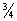0 C 60 (1,0) (1,1,0,0,0,0) 1 1 D 45 (0,0) (0,0,0,0,1,0)1

#### 2. Calculate distance matrix of each feature variable and normalize them

Now we have the coordinate of each object, but each features variables are different measurement scale. Some are binary and the others are quantitative . We cannot mix them to compute the distance because they have different type. We can only mix them after we normalize them. However, normalizing the coordinate does not solve the problem because they are still in different data type (or measurement scale). Thus, we need to calculate the distance for each feature, normalize each of them and then put them together as single distance.

In this example, we will use City block distance for quantitative variables, and Simple Matching distance for binary variables.

Distance between Time coordinates produce city-block distance matrix as in the left table below and the right table is the normalization of the distance matrix. The normalization is based on the maximum value of the distance matrix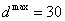. Thus, all the entries in the distance matrix are divided by the maximum distance.

 30 30 60 45 Time A B C D Time A B C D 30 A 0 0 30 15 A 0 0 1 0.5 30 B 0 0 30 15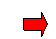B 0 0 1 0.5 60 C 30 30 0 15 C 1 1 0 0.5 45 D 15 15 15 0 D 0.5 0.5 0.5 0

Hamming distance for Mode coordinates are given in the left table below and the normalization of Hamming distance will produce Simple matching distance (right table below), that is Hamming distance divided by the total number of variables (= 2).

 (0,0) (0,1) (1,0) (0,0) Mode A B C D Mode A B C D (0,0) A 0 1 1 0 A 0 0.5 0.5 0 (0,1) B 1 0 2 1 B 0.5 0 1 0.5 (1,0) C 1 2 0 1 C 0.5 1 0 0.5 (0,0) D 0 1 1 0 D 0 0.5 0.5 0

Table below is the Simple matching distance for Activity variable. Since the value is in the range [0, 1], it is already normalized. No further normalization is necessary.

 (1,1,1,0,0,0) (0,0,0,1,0,1) (1,1,0,0,0,0) (0,0,0,0,1,0) Activity A B C D (1,1,1,0,0,0) A 0.00 0.83 0.17 0.67 (0,0,0,1,0,1) B 0.83 0.00 0.67 0.50 (1,1,0,0,0,0) C 0.17 0.67 0.00 0.50 (0,0,0,0,1,0) D 0.67 0.50 0.50 0.00

Table below is the city block distance for Satisfaction variable. Since the coordinate range is [0,1], the distance is also in the same range of [0,1]. Thus, no further normalization is necessary.

 1 0.75 1 0.25 Satisfaction A B C D 1 A 0 0.25 0 0.75 0.75 B 0.25 0 0.25 0.5 1 C 0 0.25 0 0.75 0.25 D 0.75 0.5 0.75 0

Table below is the Hamming distance for variable Playground . It is binary with only single coordinate, thus the result of the Hamming distance is already normalized.

 1 0 1 1 Playground A B C D 1 A 0 1 0 0 0 B 1 0 1 1 1 C 0 1 0 0 1 D 0 1 0 0

#### 3. Aggregate the normalized distance matrix

Now we have all the distance matrix for each features variables, the aggregation is simply weighted average of the distance . If we assumed all feature variables have the same weight, we simply sum them up and divide by 5 (= number of features variables), produce the final distance matrix as shown in the table below

 Average distance A B C D A 0.00 0.52 0.33 0.38 B 0.52 0.00 0.78 0.60 C 0.33 0.78 0.00 0.45 D 0.38 0.60 0.45 0.00

Having the distance matrix, now you can use the distance matrix for various purposes and applications such as clustering (i.e. K means clustering ) and data reduction (multidimensional scaling, Principal component analysis) and classification (LDA , Decision Tree , KNN ) and so on.

Preferable reference for this tutorial is

Teknomo, Kardi (2015) Similarity Measurement. http:\people.revoledu.comkardi tutorialSimilarity